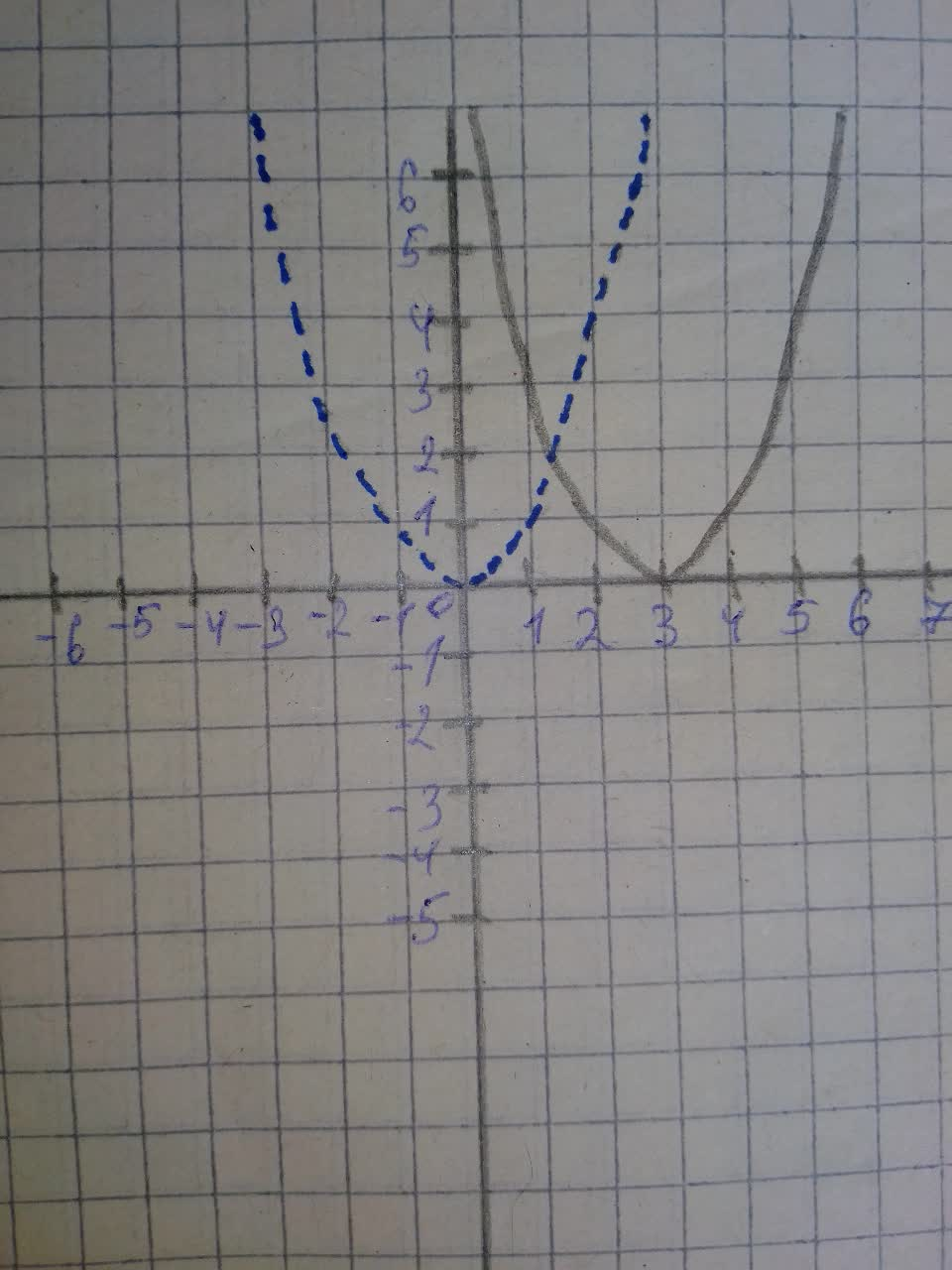Graph the function by hand, not by plotting points, y=(x-3)^{2}Clifland 2021-08-08 Answered
Graph the function by hand, not by plotting points, but by starting with the graph of one of the standard functions, and then applying the appropriate transformations.
$$\displaystyle{y}={\left({x}-{3}\right)}^{{{2}}}$$

• Questions are typically answered in as fast as 30 minutes

Solve your problem for the price of one coffee

• Math expert for every subject
• Pay only if we can solve itMargot Mill
We will start with the graph of the standard/parent function $$\displaystyle{y}={x}^{{{2}}}$$
When we shift the graph of $$\displaystyle{y}={f{{\left({x}\right)}}}$$ by k units to the right, we get the graph of $$\displaystyle{y}={f{{\left({x}-{k}\right)}}}$$
Therefore, the graph of $$\displaystyle{y}={\left({x}-{3}\right)}^{{{2}}}$$ is obtained by shifting the graph of $$\displaystyle{y}={x}^{{{2}}}$$ by 3 units to the right.
In the graph below:
The blue dashed parabola represents $$\displaystyle{y}={x}^{{{2}}}$$
The black solid parabola represents $$\displaystyle{y}={\left({x}-{3}\right)}^{{{2}}}$$